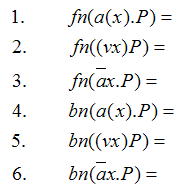# CSCI 6962 DCI Exercise 2

1. Rewrite the following expression in pi-Calculus representation

if x=y then P else Q

2. Are P|0 and P equivalent to each other? Why or why not?

3. Are P+0 and P equivalent to each other? Why or why not?

 4. Are_ a | band_ _ ( a . b + b . a )equivalent to each other?

5. Variable binding exercise ("v" denotes the restriction operator, "fn" the function to get the free variable set, and "bn" the function to get the bounded variable set):